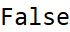# Wolfram CloudResource Object

Function Resource

# SafePrimeQ

Determines if the given number is a safe prime, or not

 ResourceFunction["SafePrimeQ"][p] gives True is p is a safe prime and False otherwise.

## Details and Options

A safe prime is a prime number of the form 2q+1, where q is also a prime.

## Examples

### Basic Examples

The prime number 23 is a safe prime, because both 23 (2·11+1) and 11 are primes:

 In:=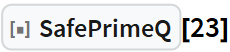Out=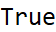The prime number 29 is not a safe prime, because 14 (29=2·14+1) is not a prime:

 In:=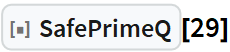Out=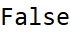The number 15 is not a safe prime, because it is not prime itself, even though 7 (from 15=2·7+1) is a prime number:

 In:=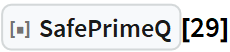Out=